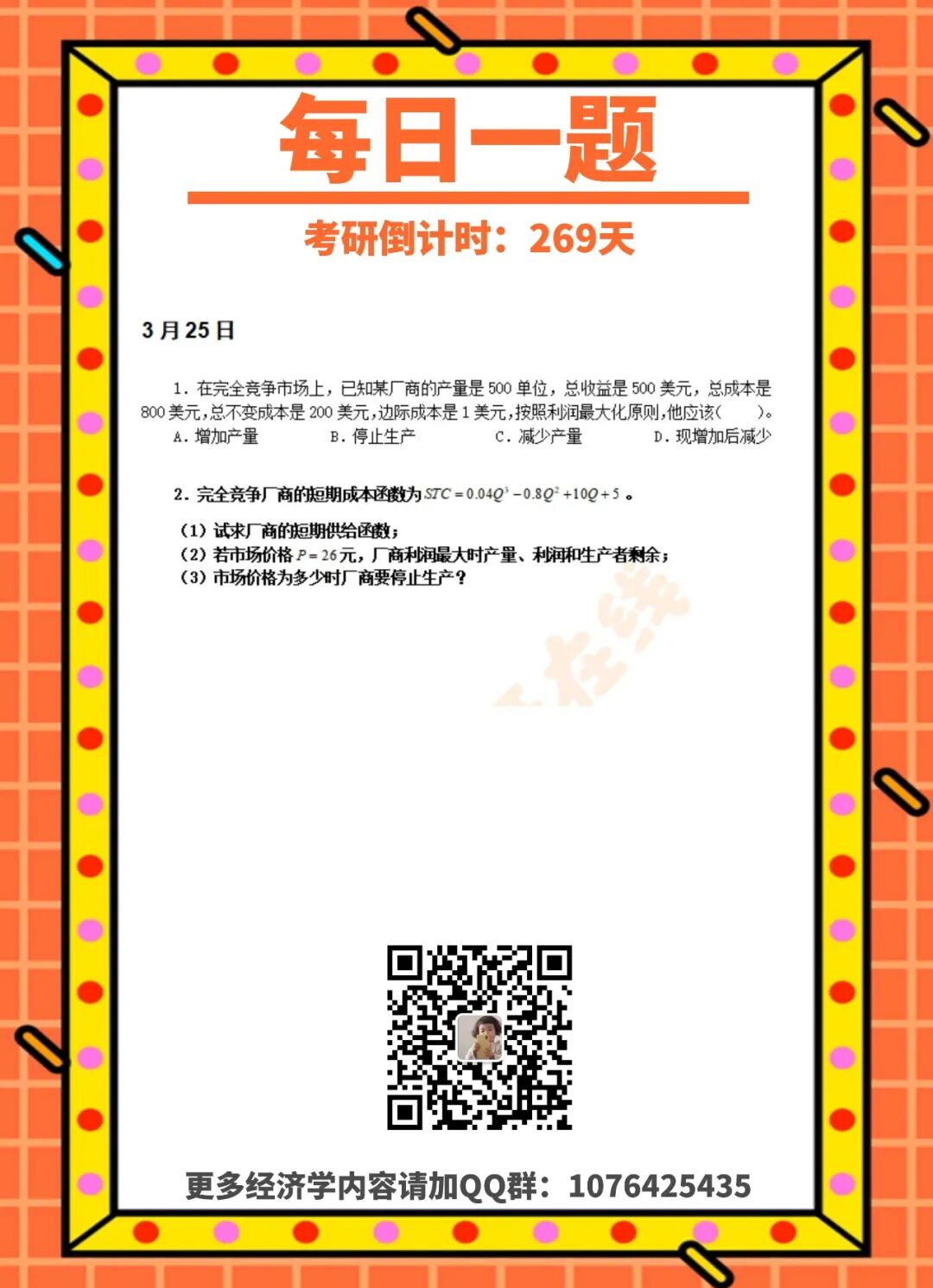晚霞龙珠白云简约黑色花卉简约米色城市粉色心情雪山绿野仙踪伦敦薰衣草加州星空

 点击蓝字关注我们每天一张经济学卡片 每天都有专业老师解析 坚持下去   成功上岸不是梦计算思考时间    解 点击空白处查看答案 1.【答案】B 【解析】厂商的平均可变成本是AVC=(800-200)/500=1.2美元。由 MC =1，P= AR = 1， 所以此时 P = AR< AVC ，所以厂商再生产下去，不仅无法获利，也不能弥补不变成本，同时还要亏损可变成本，所以应该停止生产。 2.【解析】      （1） 厂商的短期供给函数是指厂商在不同价格时愿意而且能够提供的产量，可以由厂商的边际成本曲线位于平均可变成本曲线以上的一段来表示。由已知得 AVC= 0.04Q^2-0.8Q+10,MC=0.12Q^2-1.6Q +10。令 AVC = MC ，即  0.04Q^2 -0.8Q +10 = 0.12Q ^2-1.6Q+10 解方程得Q = 10，或Q = 0（舍去）。同方法一之理，厂商的短期供给曲线为 P=  0.12Q ^2-1.6Q +10 (Q >=10)    （2）若市场价格 P =26 元，则可得厂商的利润函数为：兀 = PQ -TC = 26Q -（ 0.04Q^3-0.8Q ^2+10Q + 5 ) =-0.04Q ^3+ 0.8Q ^2+16Q - 5 要使利润极大，令 d兀/dQ= 0 ，即 d 兀/dQ=-0.12Q^2+ 1.6Q+ 16=0，解得Q =20（负值舍去），且d^2兀/dQ^2|Q=2=-0.24Q+16=-3.2您需要登录后才可以回帖 登录 | 立即注册 本版积分规则 回帖后跳转到最后一页
﻿|Archiver|手机版|小黑屋|考研狗 ( 蜀ICP备16009247号-1 )|网站地图

GMT+8, 2020-4-10 07:26